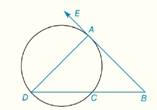Chapter 6.CR, Problem 8CRElementary Geometry For College St...

7th Edition
Alexander + 2 others
ISBN: 9781337614085

Solutions

Chapter
SectionElementary Geometry For College St...

7th Edition
Alexander + 2 others
ISBN: 9781337614085
Textbook Problem

In Review Exercises 5 to 10, B A → is tangent to the circle at point A in the figure shown. m ∠ D = 40 ° ,   m D C ^ = 130 ° ,     m ∠ B = ?To determine

To find: mB

Explanation

Theorem:

1) The measure of an angle formed when two secants intersect at a point outside the circle is one-half the difference of the measures of two intercepted arcs.

2) The measure of an inscribed angle of a circle is one-half the measure of its intercepted arc.

Calculation:

Given that

mD=40°, mDC^=130° and BA is tangent to the circle at point A.

By theorem, The measure of an inscribed angle ADC of a circle is one-half the measure of its intercepted arc mAC^.

40°=12mAC^

40°×2=12mAC^×2

80°=mAC^

Therefore, mAC^=80°

Still sussing out bartleby?

Check out a sample textbook solution.

See a sample solution

The Solution to Your Study Problems

Bartleby provides explanations to thousands of textbook problems written by our experts, many with advanced degrees!

Get Started

In Exercises 31-34, evaluate h(2), where h = g f. 31. f(x) = x2 + x + 1; g(x) = x2

Applied Calculus for the Managerial, Life, and Social Sciences: A Brief Approach

Rewrite 10x2xdx using a trigonometric substitution. a) cos10sind b) 110sincos2d c) 10cos2sind d) 10sincosd

Study Guide for Stewart's Single Variable Calculus: Early Transcendentals, 8th

For

Study Guide for Stewart's Multivariable Calculus, 8th